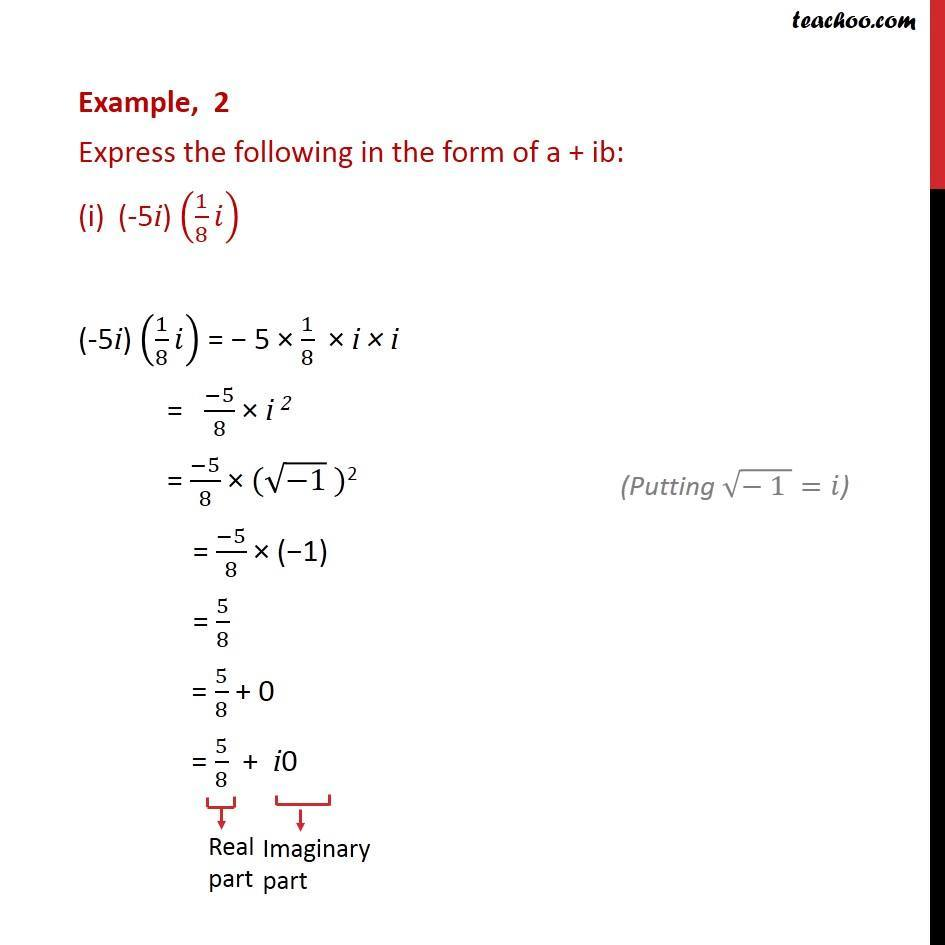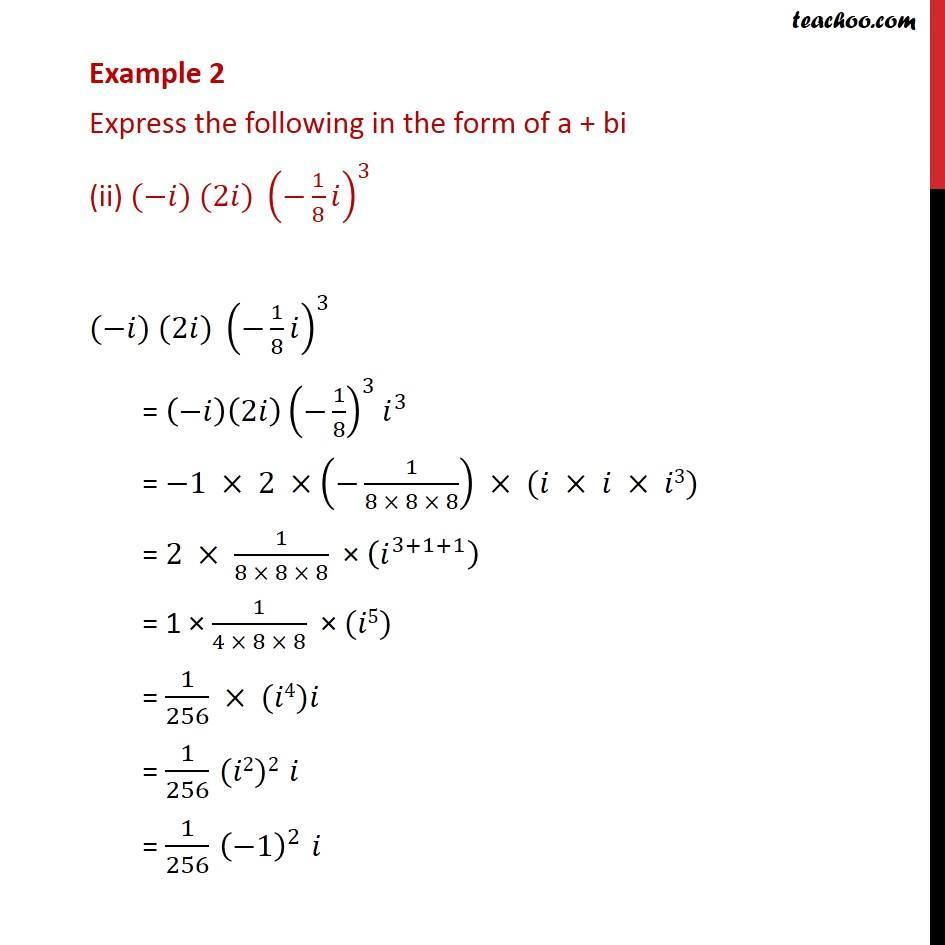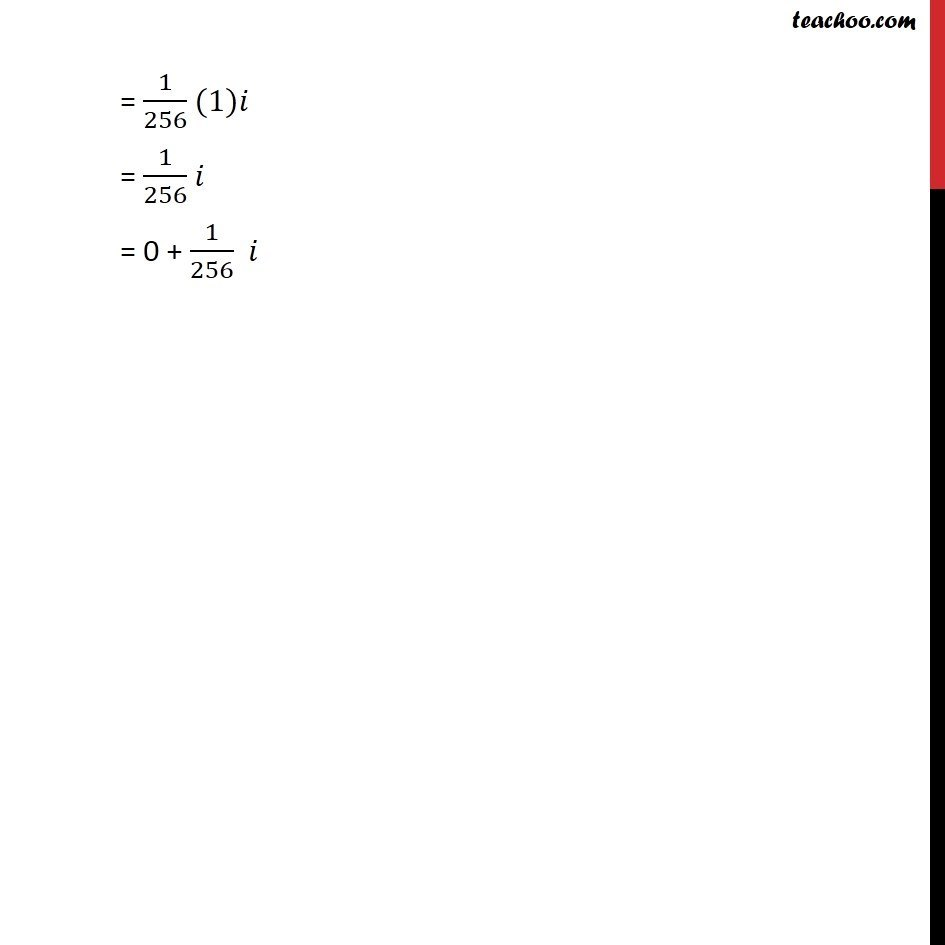Subscribe to our Youtube Channel - https://you.tube/teachoo

1. Chapter 5 Class 11 Complex Numbers
2. Serial order wise
3. Examples

Transcript

Example, 2 Express the following in the form of a + ib: (-5i) (1/8 ) (-5i) (1/8 ) = 5 1/8 i i = ( 5)/8 i 2 = ( 5)/8 ( ( 1) )2 = ( 5)/8 ( 1) = 5/8 = 5/8 + 0 = 5/8 + i0 Example 2 Express the following in the form of a + bi (ii) ( ) (2 ) ( 1/8 )^3 ( ) (2 ) ( 1/8 )^3 = ( )(2 ) ( 1/8)^3 ^3 = 1 2 ( 1/(8 8 8)) ( 3) = 2 1/(8 8 8) ( ^(3+1+1) ) = 1 1/(4 8 8) ( 5) = 1/256 ( 4) = 1/256 ( 2)2 = 1/256 ( 1)^2 = 1/256 (1) = 1/256 = 0 + 1/256

Examples